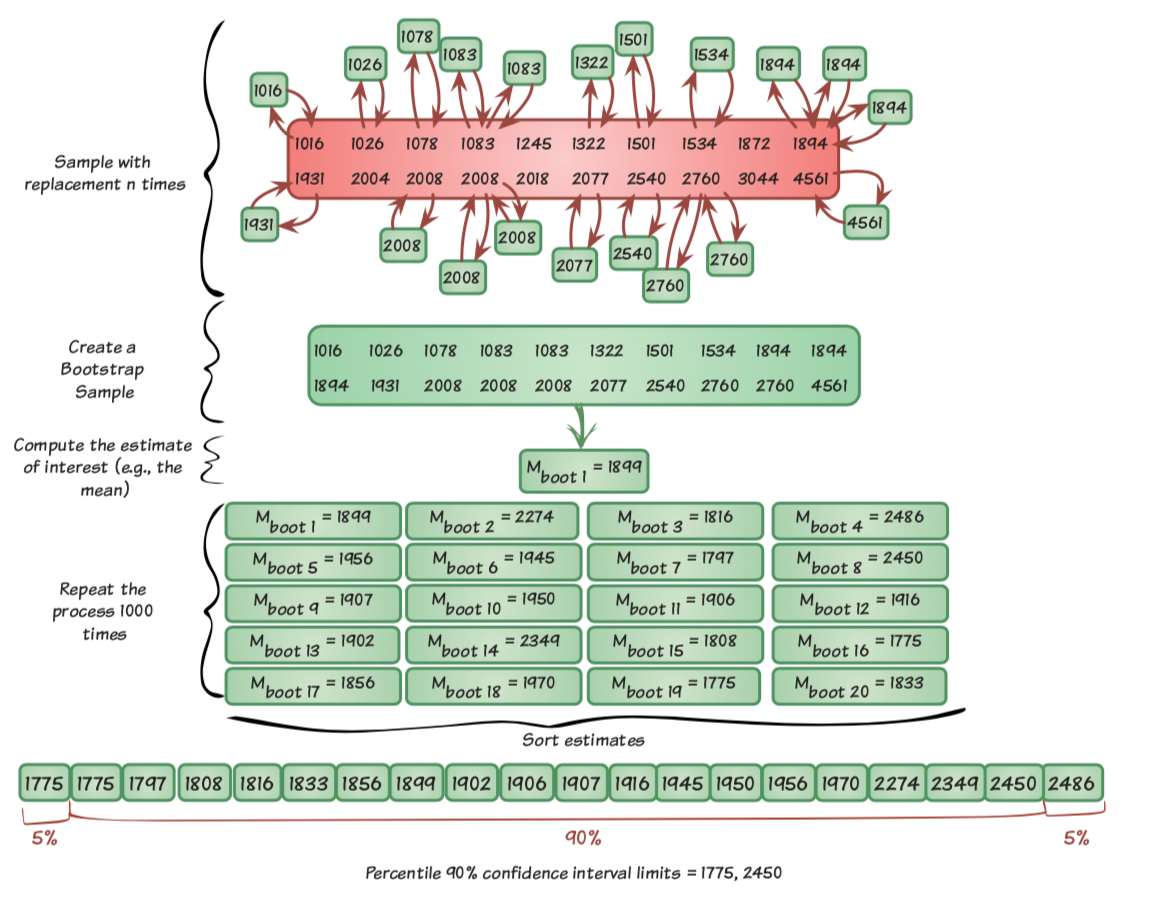# What is a parameter vector in statistical inference

## Statistics lecture

Inference allows us to infer the population from a sample. To do this, however, we must first understand why we are using sampling. Imagine you want to investigate the voting behavior of Tyroleans. Basically, you would have to interview all eligible voters in Tyrol. But this will hardly be feasible. What you can do instead is do a sample to pull. That means you only question a fraction of the Tyroleans and inquire into the voting behavior of the people interviewed (the sample). This is where inference comes in. It helps you die clarity to describe the pattern in the sample and thus be able to state with a certain degree of certainty that this pattern could also be found in the basic population (all eligible voters in Tyrol).

We start again with the idea of ​​the model. We had this in the unity of descriptive statistics already discussed: A model helps us to make a prediction about data points. We underestimate the value of some data points and overestimate the value of other data points. We call these deviations (this miscalculation) the error term.

We used the mean as an example of a model, but imagine if we didn't know how to calculate a mean, how would we find the value that would make the best prediction for our data points?

What we could do is us that optimal value approximate. To do this, we start to estimate any number and then calculate the sum of the error terms. Now we estimate another value and again calculate the sum of the error terms and then choose the estimated value which has a lower sum of the error terms. We can do this for a lot of estimates and so we can optimal value approach. We also call this process the Least squares method (we need it again for the regression analysis - unit 6).

We have now done this for the sample. It can therefore not necessarily be said that this optimal value, which we calculated on the basis of the sample, also for the basic population optimal value represents.

### 5.1 Distribution of samples

The deviation of the estimate from the sample from optimal value the basic population we call Sampling error. With one sample we underestimate the actual value in the basic population, with another sample we overestimate the actual value in the basic population. So there is one Variance in the sample. The mean of optimal values of a large number of samples, in turn, comes very close to the actual value of the population (since we overestimate ourselves with some samples and underestimate ourselves with some, this balances out again at some point). We can display the values ​​that we have calculated from the samples in a frequency distribution - with the help of a histogram. This is called the Sample distribution: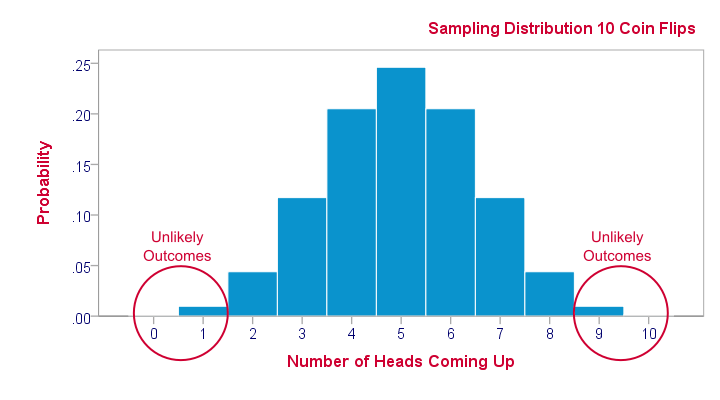In the picture you can see the sample distribution of coin flips. 10 coins were tossed per round (random sample). This process was repeated 100 times and the number of times the head was thrown was recorded. The mean in this distribution is 5, i.e. half of the throws result in heads and the other half tails. This also corresponds to the theoretical probability.

Such a sample distribution is more of a theoretical concept than that we actually measure and record it. But it helps us to understand what our sample was drawn from below.

### 5.2 standard errors

There is another point that is important in this context: Sometimes such a sample distribution is narrower, i.e. samples are more similar in their values, and sometimes further, i.e. parameters from samples are more different from one another. We can determine the width / narrowness of the sample distribution through the Standard error define. This standard error is calculated differently for each statistic. The Standard error of the mean is calculated as follows:

\ [\ sigma _ {\ bar {x}} = \ frac {s} {\ sqrt {N}} \]

Speak the Standard error of the mean is the standard deviation of the sample divided by the square root of the size of the sample (e.g. number of observations in the sample).

### 5.3 Central limit value theorem

However, the prerequisite for calculating the standard error of the mean is that our sample has many observations. Many are because with more observations a normal distribution of the observations can be achieved. If the number of observations is too small, we don't have a normal distribution but a t-distribution (comes later).

This rule lies in the Central limit theorem fundamentally. The Central Limit Theorem states that as the number of observations in samples increases, the sample distribution becomes more normal.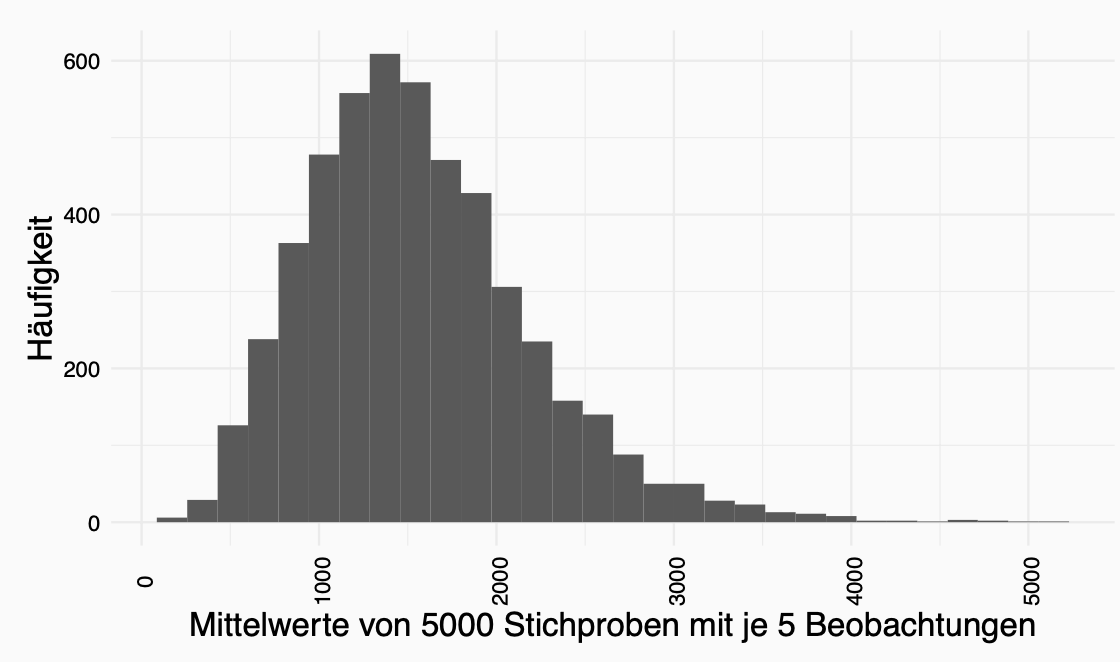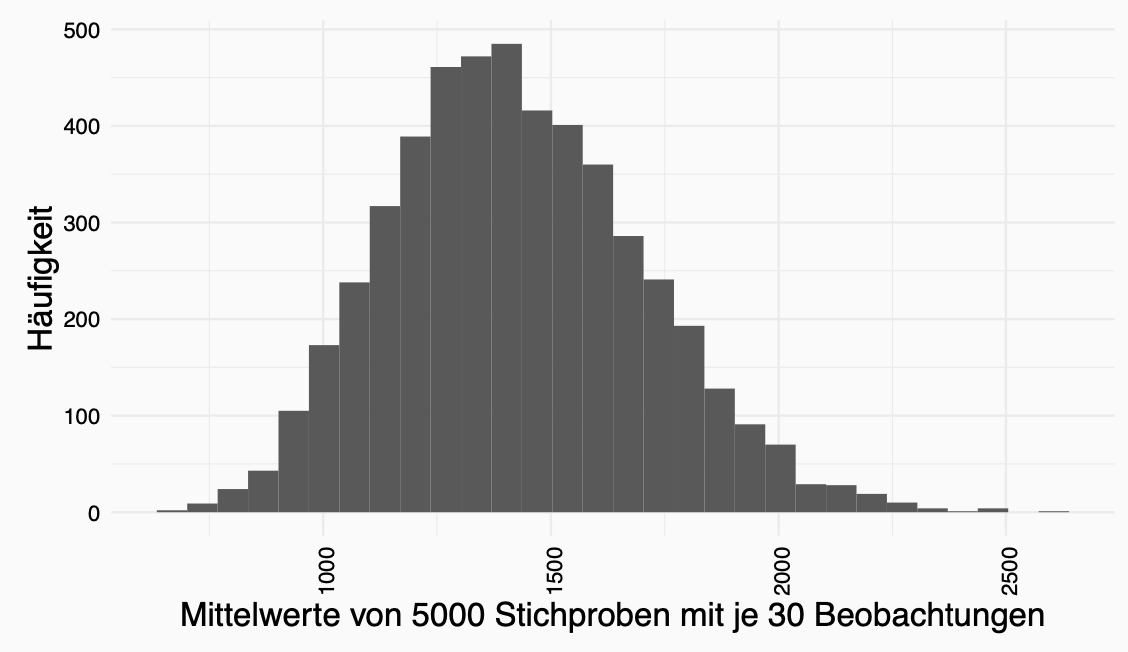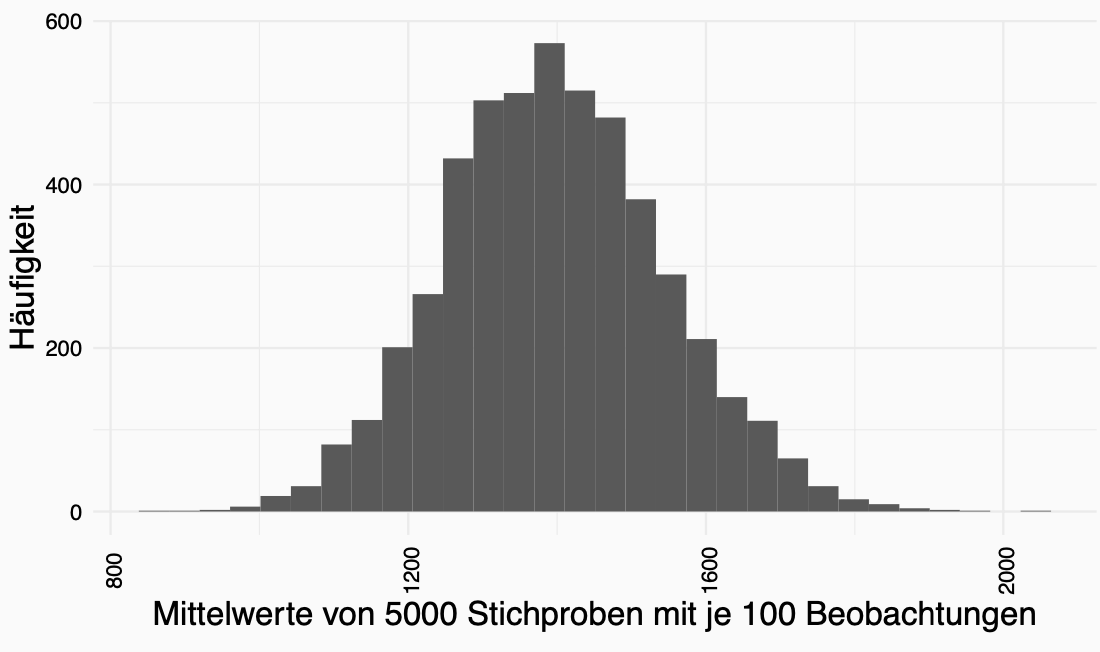Why is it all important?

We add two important points to sample distributions:

1. The width of the sample distribution informs us about the probability that a statistic of the sample is close to the parameter of the basic population. \ (\ rightarrow \) STANDARD ERROR (OF THE AVERAGE)

2. As the number of observations is increased, the distribution approaches a normal distribution. \ (\ rightarrow \) CENTRAL LIMIT VALUE SET

### 5.4 Confidence Interval

With the help of these two insights, we can Confidence intervals to calculate.

In statistics, we want to estimate certain parameters such as mean value or standard deviation based on a sample. We already got to know how we estimate these quantities in descriptive statistics. The question now arises as to how accurate these estimators are. Confidence intervals are one way of doing this. They provide an interval in which the estimated parameter lies with a given probability (typically 95%).

A Confidence interval is an interval that contains the desired parameter of a population with a given probability (i.e. confidence level or confidence level).

The idea of ​​a confidence interval is that there is a 95 percent probability that the true value of the basic population is contained in the confidence interval. So if you were to calculate 100 confidence intervals, the true value would be in 95 of the 100 confidence intervals.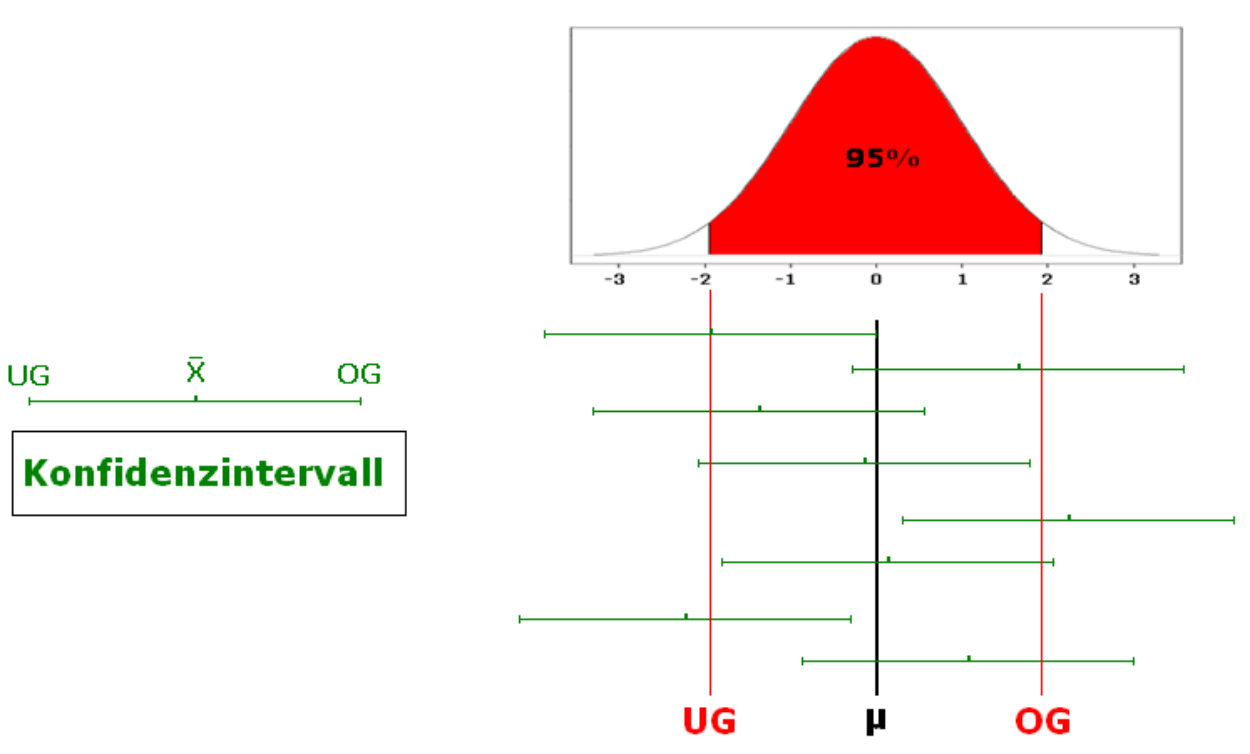Of course, we can also define probabilities other than 95 percent here. 95 percent is a common measure. We describe this probability using \ (1- \ alpha \). Alpha includes the percent that we allow not to contain the true parameter, i.e. \ (1- \ alpha \) percentage points contain the true values ​​and \ (\ alpha \) percentage points do not. This could be recorded as follows: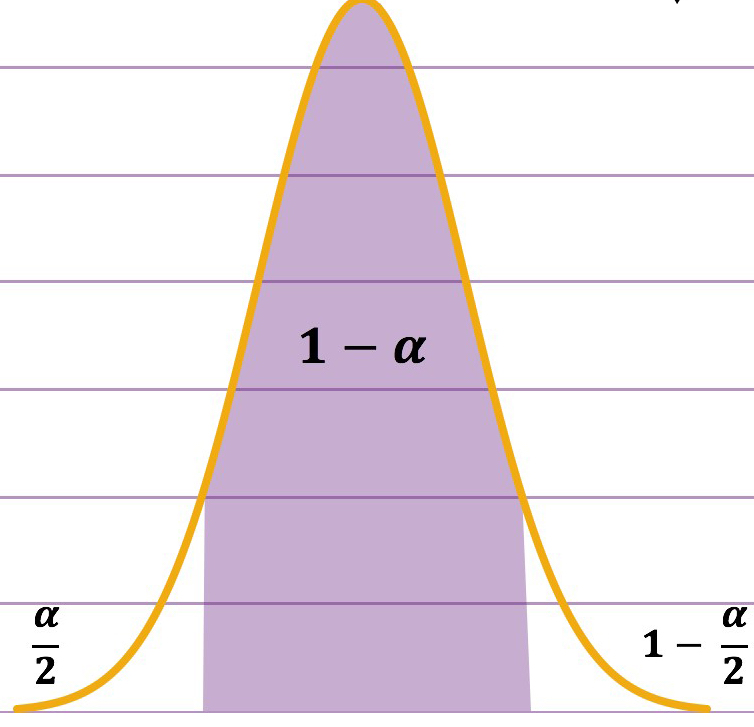For the calculation of the confidence interval we have to calculate the lower limit and the upper limit of the confidence interval. To do this, we take the following formulas:

\ (\ text {Upper limit (OG)} = \ bar {X} + SE * z_ {1- \ frac {\ alpha} {2}} \)

\ (\ text {Lower limit (UG)} = \ bar {X} - SE * z _ {\ frac {\ alpha} {2}} \)

here it is important to know that \ (SE \) den Standard error of the mean represents, \ (z _ {\ frac {\ alpha} {2}} \) describes the probability of the standard normal distribution to the point where the true values probably not yet or no longer included.

### 5.5 T-distribution

What if we have smaller samples (i.e. no normal distribution)?

We know that as the number of samples increases, the spread decreases. That is, if we have a low sample size, we have a different distribution with wider “tails” and a flatter center.

Therefore a second distribution table has been introduced, namely the Student-t-distribution. The chief brewer of Guinness, who published under the name “Student”, calculated and published this distribution.

Here we have a different table than the standard normal distribution table and we have to read the probabilities from the T table, taking into account the number of cases.

We are doing all of this Inference to be able to establish the conclusion from the sample to the population with a certain degree of certainty.

### 5.6 Robust estimators

We IGNORED two more things:

1. Runaway
2. skewed distributions

The effect of outliers is shown here. Based on the effect on the variance / standard deviation, this has a large effect on the AI. The standard deviation increases exponentially because we are squaring part of it. The standard deviation is the basis of the standard error and the standard error is used to calculate the confidence interval, so we have an effect here too.

The problem with skewed distributions is that we extract more than 5 percent of the samples that do not contain the mean.

What can we do?

1. Change the dates

2. Change the method of estimation

To change data: here it is important that we always do the same with the data. What we are interested in is the difference between the data points and as long as this remains relatively the same as long as we can manipulate data. So what can we do specifically?

transformation - Logarithm (\ (ln (x_i) \)): Reduces the effect of high outliers and positive distortion. (Attention: zero and negative values ​​cannot be logarithmized)

• Root (\ (\ sqrt {x_i} \)): Decreases high values ​​more than low values ​​and positive distortion. (Attention: only works with positive values)

• \ (\ frac {1} {x_i} \): Reduces high values ​​and positive distortion. (Attention: Reverses the values)

• \ ((x _ {\ text {Highest value}} + x _ {\ text {Lowest value}}) - x_i \): Inversion of values. (Note: is also useful in combination with one of the above methods to correct negative bias)

• For large samples, skewed distributions are no longer a big problem.

• The transformation has an effect on how we interpret the data.

• If we perform the “wrong” transformation, the consequences for the result are worse than if we do not transform.

The second way we can "modify" data is by trimming.

1. Sort data

2. Calculate the number of observations to be shortened:

\ (\ text {Number of observations} * \ text {percent} _ {trim} = \ text {The number of observations to be shortened (on each side)} \)

1. Delete observations.

2. Recalculate statistics.

But HOW MUCH of the data should you cut?

Admittedly, this decision is a bit crude. What is also crude is that we delete data. So we have other methods here.

Winsorizing is the transformation of data by limiting extreme values ​​in the statistical data to reduce the effect of potentially false outliers. It is named after the engineer and biostatistician Charles P. Winsor (1895–1951).

Instead of deleting the top and bottom, e.g. 5 percent of the data, we replace them with the next value.

Bootstrapping

BOOTSTRAPPING involves repeatedly drawing samples from the sample and calculating the statistics. Confidence intervals can be calculated from this.